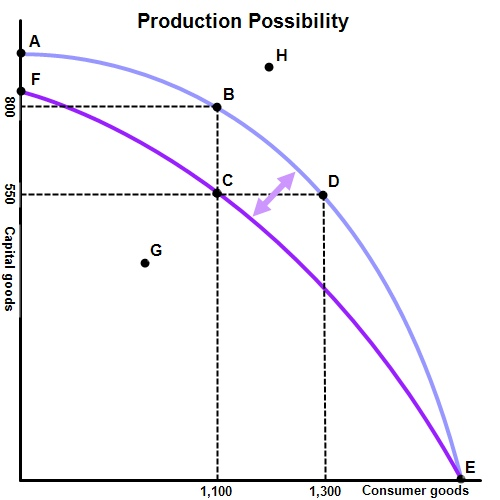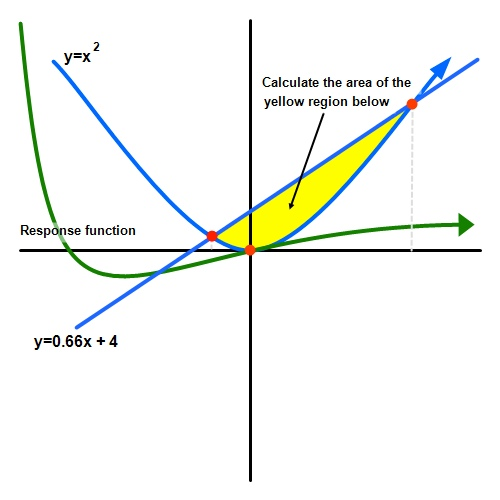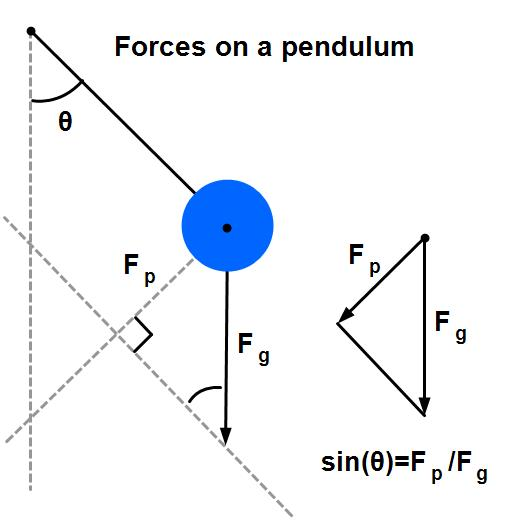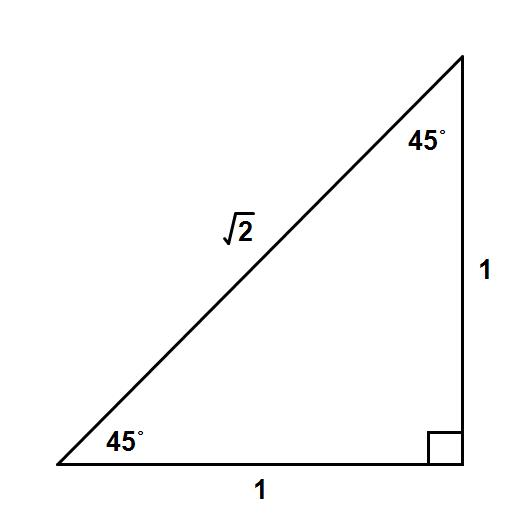# Gallery

What is a graphing program without examples of various graphs? Here are downloadable examples of graphs created with Graph Maker to help get you started. You can download each graph and edit them freely in Graph Maker.

## Economics - Supply and Demand

Sample Economics graph of supply and demand.## Economics - Production Possibility

Sample Economics graph of production possibilities frontier.## Math - Various Functions

An example of various curves modeling actual functions in Mathematics.## Chemistry - Triple Point

The graph of the triple point of a fictitious chemical formula. This graph also shows off the superscripting and subscripting available in the Graph Maker text tool.## Math - Parabola

This diagram of a parabola shows some of its characteristics in mathematical form.## Physics - Pendulum Forces

This diagram shows the forces on a pendulum as it swings to one side.## Geometry - Right Triangle

The familiar 45° right triangle showing relative lengths of each side.## Math - Spherical Trigonometry

This diagram of a sphere with a highlighted surface shows the ability of simple 2D shapes to create a nice 3D diagram.## Math - Trigonometry Unit Circle

This unit circle figure shows the derivation of all of the basic trigonometric functions.That's all folks!